Ex 9.3

Chapter 9 Class 11 Straight Lines
Serial order wise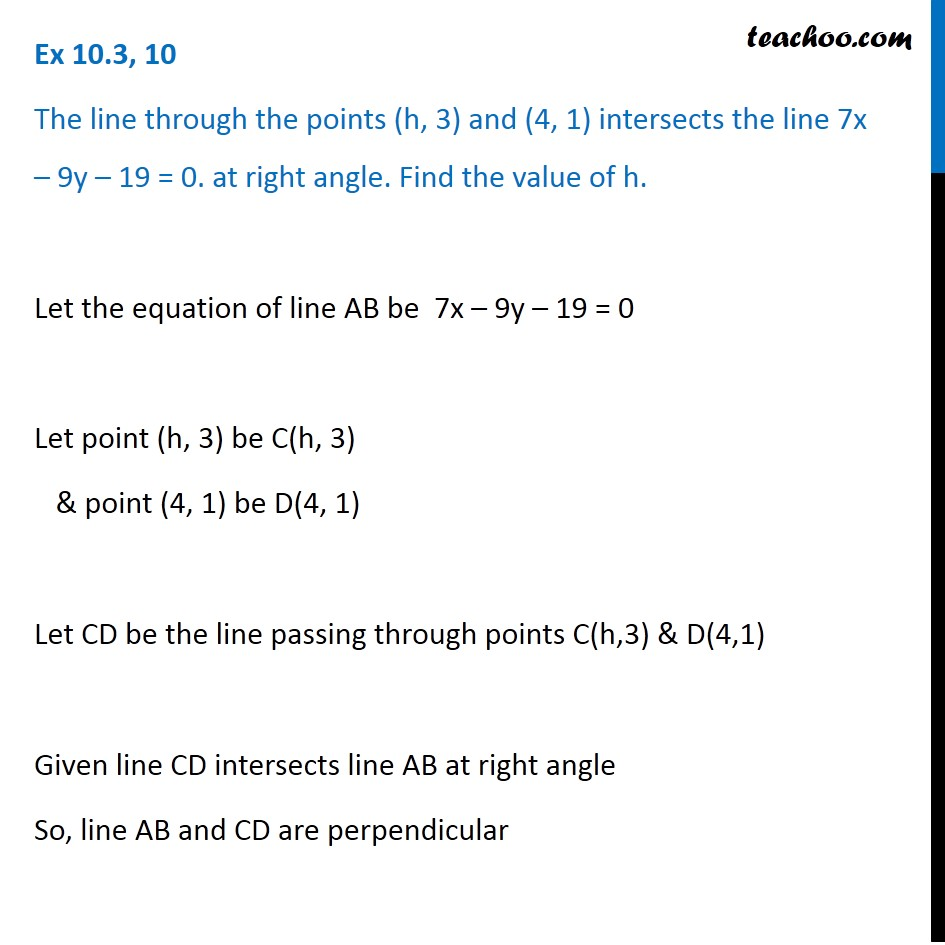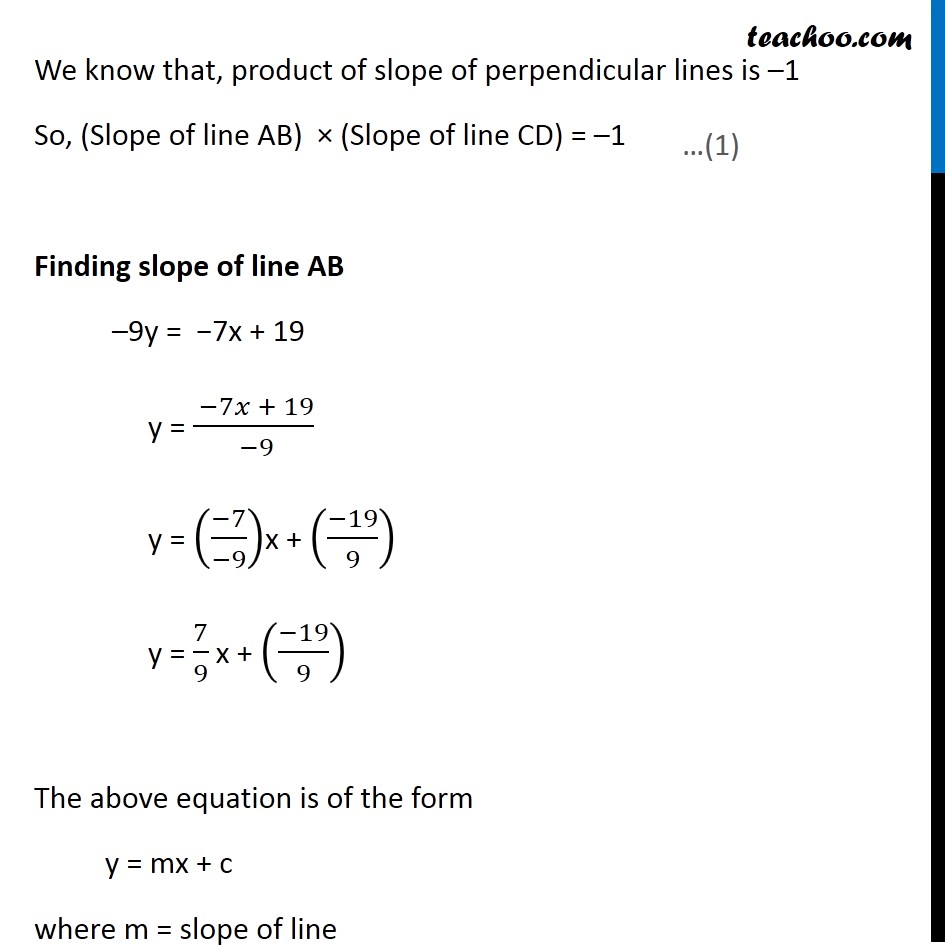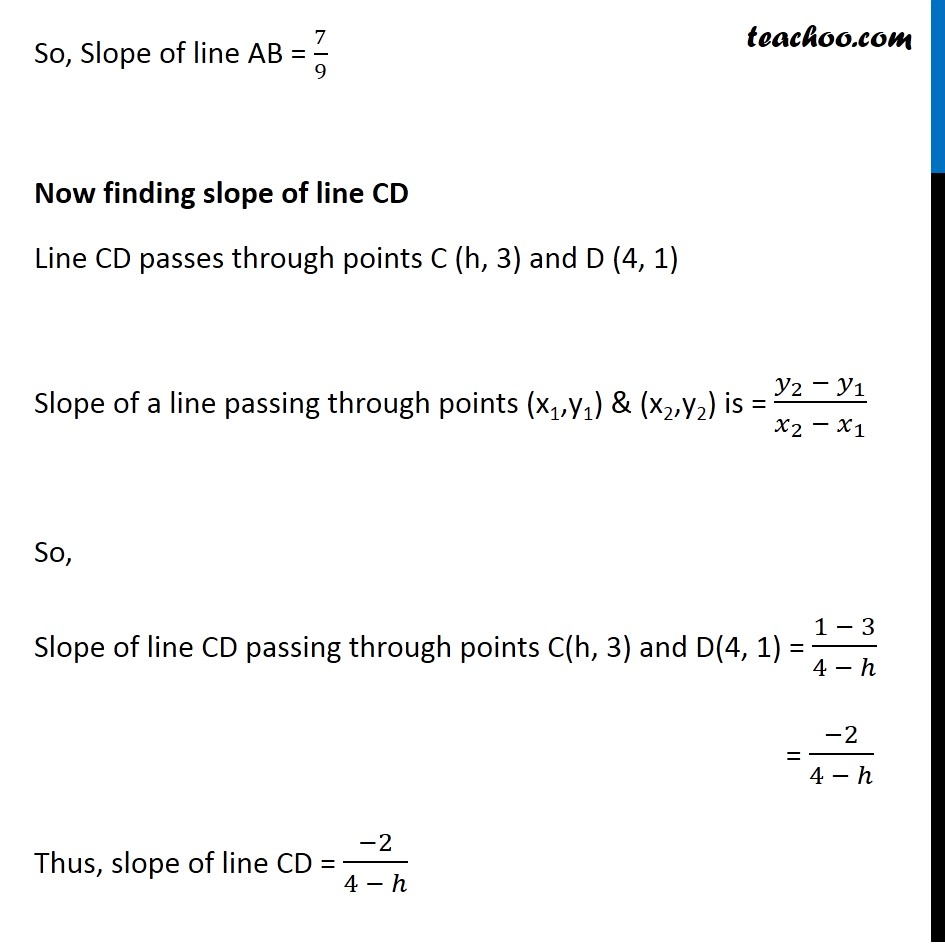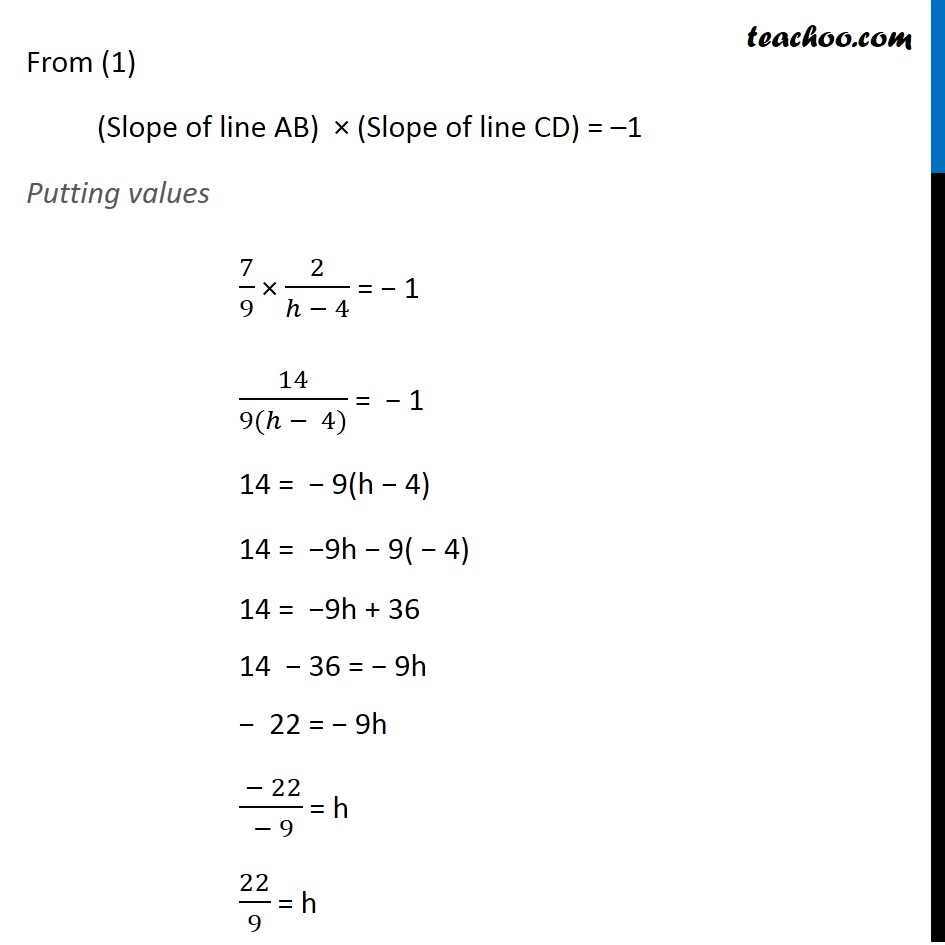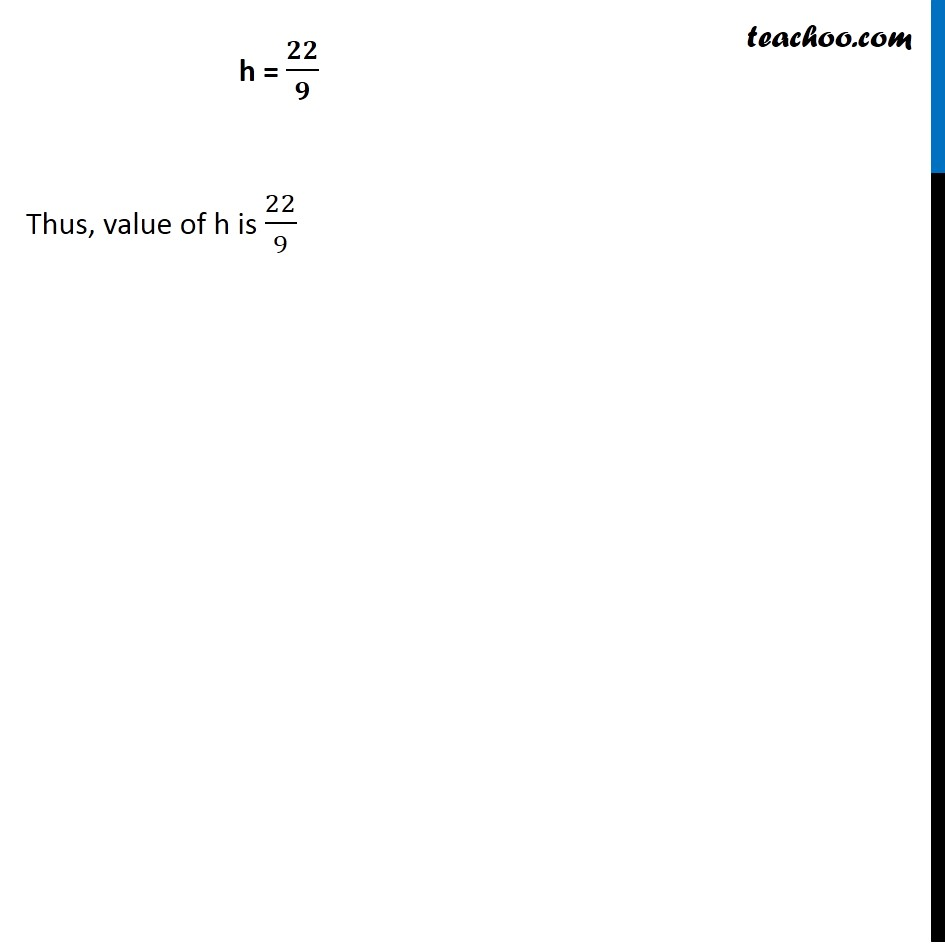Learn in your speed, with individual attention - Teachoo Maths 1-on-1 Class

### Transcript

Ex 9.3, 9 The line through the points (h, 3) and (4, 1) intersects the line 7x – 9y – 19 = 0. at right angle. Find the value of h. Let the equation of line AB be 7x – 9y – 19 = 0 Let point (h, 3) be C(h, 3) & point (4, 1) be D(4, 1) Let CD be the line passing through points C(h,3) & D(4,1) Given line CD intersects line AB at right angle So, line AB and CD are perpendicular We know that, product of slope of perpendicular lines is –1 So, (Slope of line AB) × (Slope of line CD) = –1 Finding slope of line AB –9y = −7x + 19 y = ( −7𝑥 + 19)/( −9) y = ((−7)/(−9))x + ((−19)/9) y = 7/9 x + ((−19)/9) The above equation is of the form y = mx + c where m = slope of line So, Slope of line AB = 7/9 Now finding slope of line CD Line CD passes through points C (h, 3) and D (4, 1) Slope of a line passing through points (x1,y1) & (x2,y2) is = (𝑦_2 − 𝑦_1)/(𝑥_2 − 𝑥_1 ) So, Slope of line CD passing through points C(h, 3) and D(4, 1) = (1 − 3)/(4 − ℎ) = (−2)/(4 − ℎ) Thus, slope of line CD = (−2)/(4 − ℎ) From (1) (Slope of line AB) × (Slope of line CD) = –1 Putting values 7/9 × 2/(ℎ − 4) = − 1 14/(9(ℎ − 4)) = − 1 14 = − 9(h − 4) 14 = −9h − 9( − 4) 14 = −9h + 36 14 − 36 = − 9h − 22 = − 9h ( − 22)/( − 9) = h 22/9 = h h = 𝟐𝟐/𝟗 Thus, value of h is 22/9